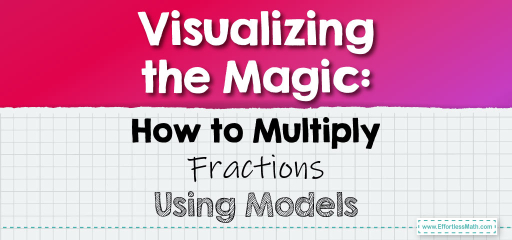# Visualizing the Magic: How to Multiply Fractions Using Models

Multiplying fractions is a fundamental skill in mathematics, and while the process is straightforward, visualizing it can provide a deeper understanding.By using models, we can see how fractions multiply to create a new value. In this blog post, we’ll walk through a step-by-step guide on multiplying fractions using visual aids.

## Step-by-step Guide:

1. Basics of Multiplying Fractions:

When multiplying fractions, we multiply the numerators together for the new numerator and the denominators together for the new denominator.

2. Setting Up Models:

Draw models (like rectangles) for each fraction. Shade the portion represented by the fraction. For instance, for $$\frac{1}{3}$$, shade one-third of the rectangle.

3. Visualizing the Multiplication:

Overlay the models to see the shared shaded area. This area represents the product of the two fractions.

4. Calculating the Product:

Multiply the numerators together for the new numerator and the denominators together for the new denominator. Simplify the fraction if possible.

### Example 1:

Multiply $$\frac{2}{3}$$ by $$\frac{3}{4}$$ using models.

Solution:

The product’s numerator is $$2 \times 3 = 6$$ and the denominator is $$3 \times 4 = 12$$. So, $$\frac{2}{3} \times \frac{3}{4} = \frac{6}{12}$$, which simplifies to $$\frac{1}{2}$$.

The Absolute Best Book for 5th Grade Students

### Example 2:

Multiply $$\frac{1}{5}$$ by $$\frac{2}{7}$$ using models.

Solution:

The product’s numerator is $$1 \times 2 = 2$$ and the denominator is $$5 \times 7 = 35$$. So, $$\frac{1}{5} \times \frac{2}{7} = \frac{2}{35}$$.

### Practice Questions:

1. Multiply $$\frac{3}{4}$$ by $$\frac{2}{5}$$ using models.

2. Multiply $$\frac{1}{6}$$ by $$\frac{4}{9}$$ using models.

3. Multiply $$\frac{5}{8}$$ by $$\frac{3}{7}$$ using models.

A Perfect Book for Grade 5 Math Word Problems!

1. $$\frac{3}{10}$$

2. $$\frac{2}{9}$$

3. $$\frac{15}{56}$$

The Best Math Books for Elementary Students

### What people say about "Visualizing the Magic: How to Multiply Fractions Using Models - Effortless Math: We Help Students Learn to LOVE Mathematics"?

No one replied yet.

X
51% OFF

Limited time only!

Save Over 51%

SAVE $15 It was$29.99 now it is \$14.99